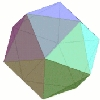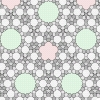# Plus Advent Calendar Door #13: Pure pleasure

Monday mornings can be messy and horrible, so to purify your mind we give you some pure mathematical favourites:The prime number lottery
Marcus du Sautoy begins a two part exploration of the greatest unsolved problem of mathematics: the Riemann Hypothesis. In the first part, we find out how the German mathematician Gauss, aged only 15, discovered the dice that Nature used to chose the primes.

And here is the second part of the series, The music of the primes.An enormous theorem: the classification of finite simple groups
Enormous is the right word: this theorem's proof spans over 10,000 pages in 500 journal articles and no-one today understands all its details. So what does the theorem say? Here is a short and sweet introduction.The trouble with five
Squares do it, triangles do it, even hexagons do it — but pentagons don't. They just won't fit together to tile a flat surface. So are there any tilings based on fiveness? This article takes us through the five-fold tiling problem and uncovers some interesting designs in the process.Gödel and the limits of logic
When Kurt Gödel published his incompleteness theorem in 1931, the mathematical community was stunned: using maths he had proved that there are limits to what maths can prove. This put an end to the hope that all of maths could one day be unified in one elegant theory and had very real implications for computer science. This article describes Gödel's brilliant work and troubled life.Omega and why maths has no TOEs
Gregory Chaitin explains why he thinks that Gödel's incompleteness theorem is only the tip of the iceberg, and why mathematics is far too complex ever to be described by a single theory.Cantor and Cohen: Infinite investigators Part I and Part II
What's the nature of infinity? Are all infinities the same? And what happens if you've got infinitely many infinities? These two articles explore how these questions brought triumph to one man and ruin to another, venture to the limits of mathematics and find that, with infinity, you're spoilt for choice.

Back to the Plus Advent Calendar